# High School Chemistry : Acid-Base Chemistry

## Example Questions

← Previous 1 3 4 5 6 7 8 9 10

### Example Question #1 : Definitions Of Acids And Bases

What acid-base definition describes a compound that accepts an electron pair?

Brønsted-Lowry base

Lewis acid

Arrhenius acid

Lewis base

Lewis acid

Explanation:

There are three principle definitions for acids and bases.

The Arrhenius definition is the simplest, and states that acids are compounds that increase proton concentration in solution, while bases are compounds that increase hydroxide concentration in solution. Arrhenius acids will always contain hydrogen and Arrhenius bases will always contain hydroxide groups.

Brønsted-Lowry definitions center on proton donation. Brønsted-Lowry acids are species that donate protons, and Brønsted-Lowry bases are compounds that accept protons.

Lewis acids and bases refer to the donation or acceptance of an electron pair. Lewis bases donate an electron pair, and Lewis acids accept an electron pair.

### Example Question #2 : Acid Base Chemistry

Which of the following concentrations indicates a basic solution?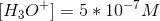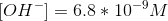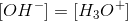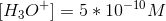Explanation:

An acidic solution will always have a hydronium concentration greater than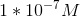. A basic solution will always have a hydroxide concentration greater than. If the hydroxide concentration is equal to the hydronium concentration, the solution is neutral.

The opposite of the above conditions can also be noted. If the hydronium concentration is less than, then the solution will be basic. An example of this is a solution where the hydronium ion concentration is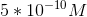. Since this value is far less than, this will be a basic solution.

### Example Question #3 : Acid Base Chemistry

Hydrochloric acid (HCl) is which of the following?

A weak acid

A neutral salt

A strong acid

A strong base

A weak base

A strong acid

Explanation:

Strong acids and bases dissociate completely into ions when placed in an aqueous solution. In contrast, weak acids and bases do not completely dissociate.

There are only six strong acids: perchloric acid (HClO4), hydroiodic acid (HI), hydrobromic acid (HBr), hydrochloric acid (HCl), nitric acid (HNO3), and sulfuric acid (H2SO4).

### Example Question #4 : Acid Base Chemistry

How does the Brønsted-Lowry theory of acids and bases describe acids?

Brønsted-Lowry acids are acids that dissociate 100% in a solution

Brønsted-Lowry acids are proton acceptors when dissolved in water

Brønsted-Lowry acids are electron acceptors when dissolved in water

Brønsted-Lowry acids are proton donors when dissolved in water

Brønsted-Lowry acids are any compound that dissociates in water to yield a proton

Brønsted-Lowry acids are proton donors when dissolved in water

Explanation:

There are three primary classifications of acids and bases.

Arrhenius acids yield protons when dissolved in solution, while Arrhenius bases yield hydroxide ions.

Brønsted-Lowry acids are protone donors, while Brønsted-Lowry bases are proton acceptors.

Lewis acids are electron acceptors, while Lewis bases are electron donors.

An acid that dissociates completely in solution is considered a strong acid due to its high Ka value.

### Example Question #5 : Acid Base Chemistry

By definition, a Lewis base is any species that __________.

has a pH below 7

accepts a pair of electrons from a Lewis acid

removes hydrogens from a solution

donates a pair of electrons to a Lewis acid

always produces a gas as a product

donates a pair of electrons to a Lewis acid

Explanation:

The most common Lewis bases are anions, and therefore, have unpaired valence electrons which may be donated to Lewis acids. The concept of donating and/or accepting hydrogens refers to the Bronsted-Lowry definition of acids. A Bronsted-Lowry acid donates hydrogens.

### Example Question #6 : Acid Base Chemistry

All acids have which phase label?

(aq)

(g)

(l)

(s)

(r)

(aq)

Explanation:

Acids are soluble in water substance, but remember, only water gets the liquid (l) phase label. Since acids are almost always dissolved in water, we use the (aq) subscript at the end of the chemical formula to indicate its phase.

### Example Question #7 : Acid Base Chemistry

According to the Lewis definition, acids are __________.

hydrogen donors

hydroxide acceptors

electron pair donors

electron pair acceptors

hydrogen acceptors

electron pair acceptors

Explanation:

According to the Lewis definition, acids are electron pair acceptors.

### Example Question #8 : Acid Base Chemistry

What is the Lewis definition of bases?

accept hydrogen ions

electron pair acceptors

increase the concentration of hydroxide ions in a solution

increase the concentration of hydrogen ions in a solution

electron pair donors

electron pair donors

Explanation:

According to the Lewis definition, bases are electron pair donors.

### Example Question #1 : Acids And Bases

What does amphoteric mean?

A substance cannot act as an acid or a base

substance has a high concentration of neutrons

A substance that is highly acidic

substance is highly basic

A substance can act either as an acid or a base

A substance can act either as an acid or a base

Explanation:

An amphoteric substance is one that can act either as an acid or a base. Amino acids are good examples of amphoteric substances; they have a carboxylic acid group that can be acidic, and an amino group that can be basic.

### Example Question #10 : Acid Base Chemistry

What is a diprotic acid?

An acid with 3 ionizable hydrogens

An acid with 2 ionizable hydrogens

An acid with 1 ionizable hydrogen

A really weak base

A really strong base

Acids have hydrogen ions and balanced charges, "di" tells us that the acid in question has two potential hydrogen ions, which can be ionized and turned into protons (protic). These acids, like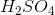can ionize once, yielding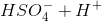then again, yielding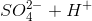.